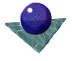Pose Estimation:
IntroductionPose estimation is the process of determining the translation and rotation of an object in one coordinate frame with respect to another coordinate frame. There are many variations on this basic problem depending primarily on the nature of the objects and the coordinate spaces. In this section, we shall consider only rigid objects; for convenience we shall assume that we have a preformed model of the object in a database and we wish to determine the position of that object in an image on the basis of matched features. However, we could equally apply the approach to find the rotation and translation of a rigid body in one frame of an image sequence with respect to a previous frame, for example.

In the simplest pose estimation problem, the scene and model data are two dimensional, for example when flat 3D objects are viewed normally on a plane. In that case, the pose can be expressed in terms of one rotational and two translational parameters. As a more general case, we shall consider the estimation of pose of a 3D object in 3D space. This situation arises commonly when orthogonal depth data is acquired by a rangefinder and compared to a CAD or other 3D model. The other case we shall consider is the pose of an object in a 2D perspective projection when compared to a 3D model. This situation arises commonly when we acquire an intensity image with a single video camera.Comments to: Sarah Price at ICBL.